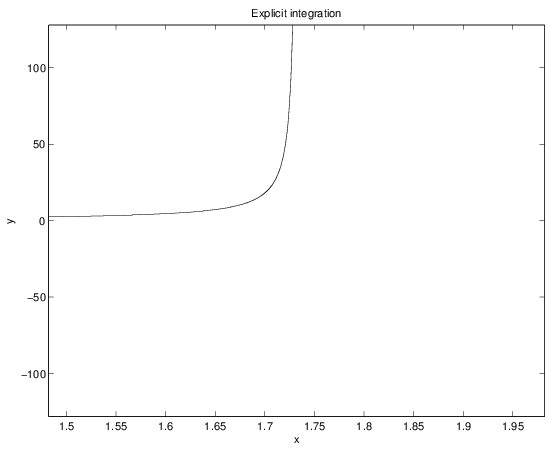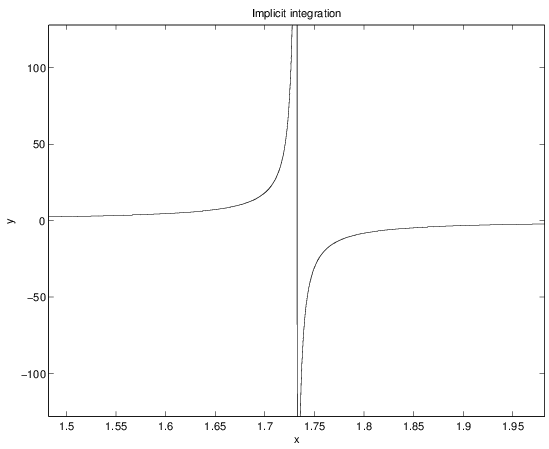# How to: Zero Delay State Variable Filter Part 2

In this part we will define what implicit integration is and develop the tools to solve differential equations with it.

### Implicit vs. Explicit

Explicit integration of difference equations relies on information that is derived from the current and past steps. This seems like a very intuitive and natural idea - surely all dynamics we observe in our physical reality happen in this causal way. One thing follows from the past things - this is taken for granted by everyone.

In practice however, this idea does not work in all situations as will be shown in the next sections. In contrast to explicit integration, implicit integration uses information on the next state as well as the current and past states. But then there is an obvious problem - where do you get information on the next state if you’re not there yet? Naively, this seems absurd.

As discussed before in the first part analog circuitry seems to solve this issue naturally, there is no need for explicit integration schemes since the feedback paths are zero delay. How can a deterministic finite state computer compete with this, surely it must be impossible to achieve this with digital filters!

### Worst case nonlinear differential equation

Let us now compare implicit and explicit integration in detail with a nasty example that has an exact solution.

#### Exact closed-form solution

The nonlinear differential equation

has an exact closed-form solution by separating the variables.

For initial conditions $x=1, y(1)=1$, we get

It’s obvious that there is a singularity at $\sqrt{3}$ for this solution:#### Solution by explicit integration

Let’s build an algorithm to solve this nonlinear differential equation by explicit integration. Euler method will suffice to make the point clear.

The derivative is evaluated as $y’(x)=f(x, y)=xy^2$ and the state integrating algorithm is

The pseudocode for the integration is simply

With initial conditions $x=1, y(1)=1$ and $h=0.0001$ the explicit integration gets us the result in the next figure.It’s obvious that something went horribly wrong! The integration can’t resolve the singularity and instead just shoots up to infinity.

Noteworthy, that analog computation wouldn’t do any better. The integration would just hit the positive voltage rail instead of reaching to infinity exponentially. Even if an analog circuit can be called a zero delay feedback system, it is still working with explicit integration.

Enter implicit integration.

#### Solution by implicit integration

The usual starting point for implicit integration is the trapezoidal rule:

Since $y’=f(x, y)=xy^2$, we get

The left-hand side of the equation now contains a quadratic function of the $y_{i+1}$ term, which you need to solve to advance to the next integration step. This is where the term implicit comes from - you do not have an explicit function to calculate the next step from.

We need to attack the equation with a tool developed by the foremost alchemist/occultist to have ever lived - our friend Sir Isaac Newton. The plan is to arrange the equation as an quadratic function of $y_{i+1}$ and use the Newton’s method to iterate towards a numerical approximation.

The right-hand side of the equation can be simplified as a constant $A_i=y_{i} + \frac{1}{2} h x_{i} y_{i}^2$ and

Further, swapping the variables $x=y_{i+1}, a=x_{i+1}$, we get the function

and it’s derivative

Newton’s method can now be constructed as

to compute $x=y_{i+i}$ to arbitrary approximation.

The pseudocode for the implicit solver is then

The iteration in the loop takes from 3 to 8 cycles in the worst case to reach the maximum floating point accuracy, which should be more than enough for practical purposes.

The result is the following:If the timestep $h=0.0001$ is chosen to be small enough, the integration now succesfully captures the singularity, unlike explicit integration.

Obviously, there are errors near the point of singularity $\sqrt{3}$ (which can be succesfully minimized using adaptive step-lengths) but it is truly a show of force for the implicit methods to take down the absolute worst you can throw at an integration algorithm and come out of it unscathed.

We will finally conclude the quest for the zero-delay feedback state-variable filter in the next part, having first built up the tools and concepts to deal with it.

Happy hacking, as always!